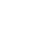## How can I plot a model-fitted spectrum in photon flux units (photons s-1 cm-2 keV-1)?

In Sherpa 4.9, model-fitted source spectra are plotted in units of counts s-1keV-1 (or per channel or wavelength). This is because Sherpa convolves an assumed source model in photon flux units with the instrument response functions to get a model-predicted flux in counts s-1keV-1, so that this model may be compared to a data set in counts. To plot in photon flux units (photons s-1 cm-2 keV-1) would require doing things the other way around - it requires deconvolving the data using the RMF and ARF instrument responses and comparing it to a model which is unconvolved (i.e., the model representation of source flux before it passes through the detector).

The following set of Sherpa 4.9 commands may be used to plot a model-fitted source spectrum in photon flux units. (To unfold a source spectrum independent of a model, see Sherpa Plotting FAQ #3.)

(Please note that the following produces only an approximation of the deconvolved source data.)

Referencing the example data used in the Sherpa thread "Introduction to Fitting PHA Data":

```sherpa> plot_data()
sherpa> plot_source()

sherpa> xx = get_fit_plot().dataplot.x
sherpa> dd = get_fit_plot().dataplot.y
sherpa> ee = get_fit_plot().dataplot.yerr
sherpa> mm = get_fit_plot().modelplot.y

sherpa> src = get_source()(xx)
["line.style", "0", "err.style", "line",
"symbol.style", "4","symbol.size", "3",
"symbol.fill", "false"])
sherpa> set_plot_ylabel("Photons sec^{-1} cm^{-2} kev^{-1}")
sherpa> set_plot_xlabel("Energy (keV)")

sherpa> plot_source(overplot=True)
sherpa> log_scale()
```

First, data is loaded (with load_pha) and plotted with plot_data, then a source model is defined (with set_source) and plotted with plot_source. The get_fit_plot function is then used to store the x and y arrays of the data plot to variables 'xx' and 'dd', respectively; the data y errors to variable 'ee'; and the y array of the model plot to variable 'mm'. Next, the get_source function is used to store the unconvolved model values to the variable 'src' (while 'mm' contains the convolved model values). Finally, the ChIPS function add_curve creates a plot of photon flux (photons s-1 cm-2 keV-1) versus energy (keV), and the 'overplot' option of plot_source is used to add the convolved model to the plot.

For XSPEC source models (those that begin with xs), the energy bin-width needs to be taken into account. The get_source() command above should be replaced with

```sherpa> bw = xx[1:]-xx[0:-1]
sherpa> bw = numpy.append(xx-xx,bw)
sherpa> src = get_source()(xx)/bw
```

The plotted values in Figure 1 represent the ratio of the source count rate to the convolved model count rate, times the unconvolved model (photons s-1 cm-2 kev-1).Figure 1. Model-fitted source spectrum plotted in photon flux units, in a linear scale on the left and log scale on the right.

Background:

The primary function of software packages like Sherpa is to solve the integral equation (instrument response) which relates the total counts produced in a given pulse-height bin in a spectrum to the incident source spectrum. Therefore, once you have a pulse-height spectrum and the appropriate RMF and ARF instrument response, you can use Sherpa to fold the source data through an assumed model to determine the "true" source flux (see also the Sherpa function 'calc_photon_flux, which returns non-integrated flux in units of photons s-1 cm-2 keV-1 when supplied with a single energy value, and when working in energy space with set_analysis("energy")).

Though be warned that Sherpa has no explicit knowledge of data or model units; the units displayed with computed fluxes are defaults, generally correct for standard analyses of 1-D PHA energy/wavelength spectra (XSPEC-like analyses). They may be incorrect for non-standard analyses, or for analyses of 2-D spatial images with exposure maps, etc. The correct units can be determined by working backwards from the data, taking into account the exposure time, the units of the instrument model, the bin units, etc.